## 当我说我想了解一下线性回归。。。。

### 当我说我想了解一下线性回归。。。。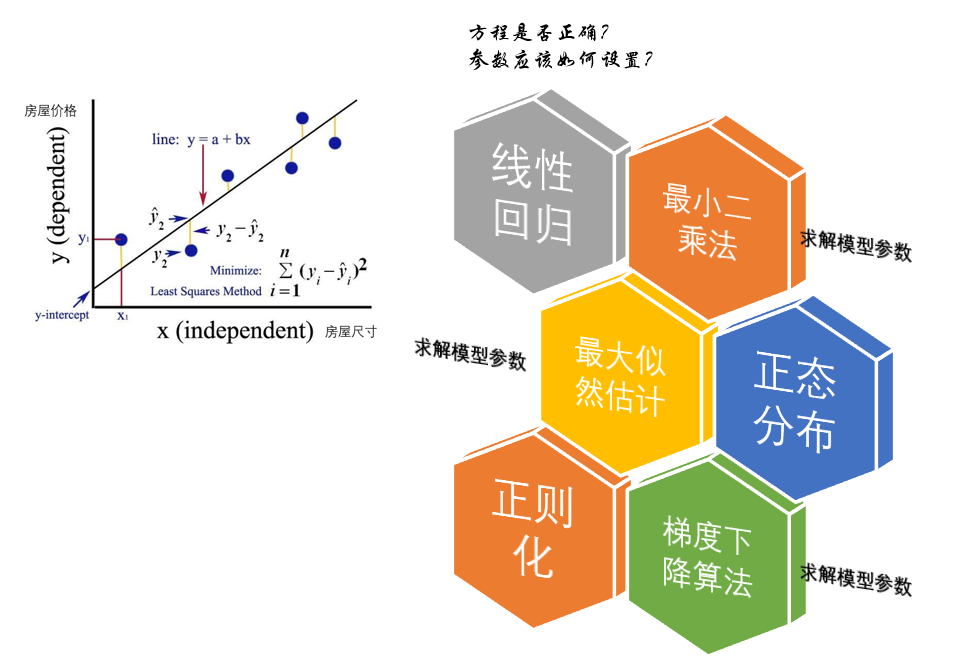### 线性回归算法

1线性回归模型介绍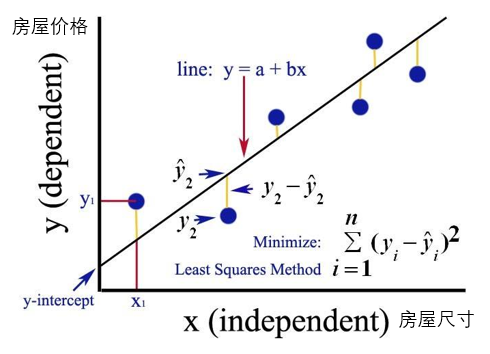$y=a+bx$

$L(a,b)=\sum_{i}^{n}(y_{i}-(a+b_{x_{i}}))^{2}$

### 最小二乘法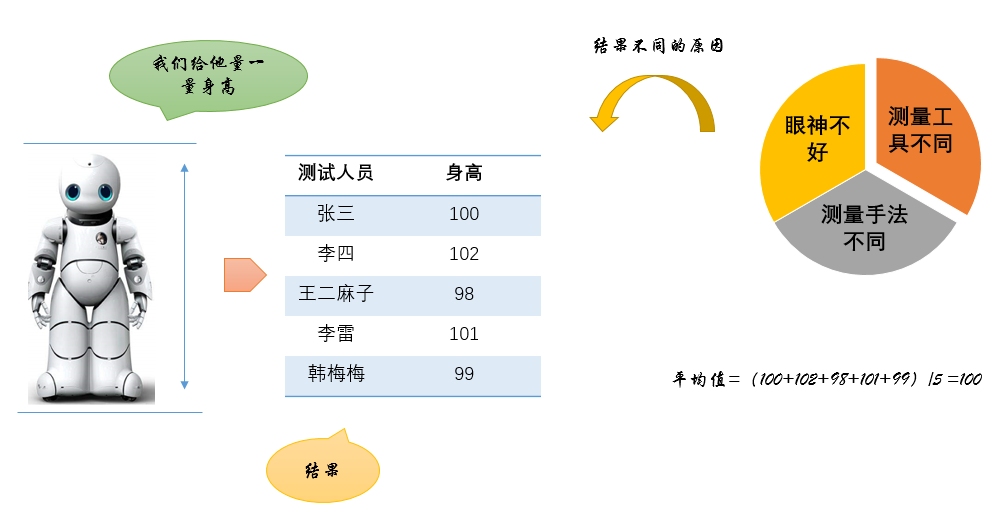1，这些人眼神不好
2，这些人用的测量工具不同
3，这些人的测量手法不同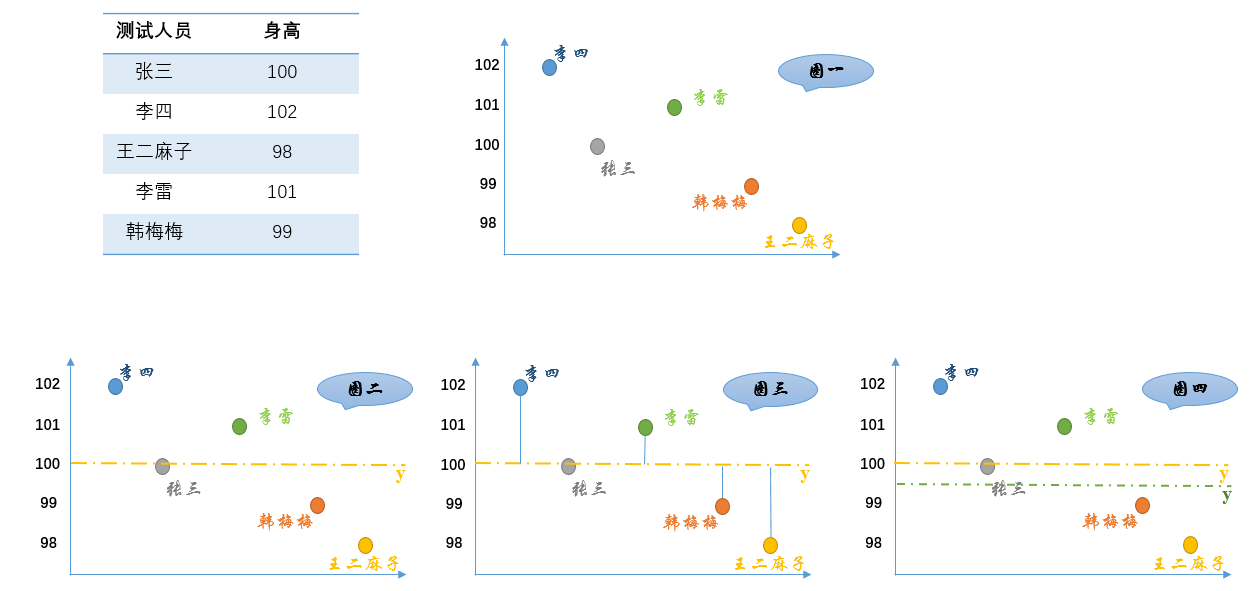1,首先，把测试得到的值画在笛卡尔坐标系中（图一）
2,其次，把要猜测的线段长度的真实值用平行于横轴的直线来表示（因为是猜测的，所以用虚线来画），记作 $y$（图二）
3,每个点都向 $y$做垂线，垂线的长度就是 $|y-测量值|$，也可以理解为测量值和真实值之间的误差（图三）
4，这里的 $y$是猜测的值，因此可以不断变化（图四）

$y=(张三+李四+王二麻子+李雷+韩梅梅）/5$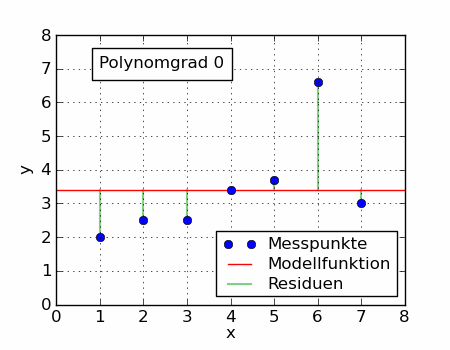### 最大似然估计法

$L(x)=p(\varepsilon _{1})+p(\varepsilon _{2})+p(\varepsilon _{3})...=\sum_{i}^{n}p(\varepsilon _{i})$

$\frac{d}{d x }lnL(x )=0$

$f(x;\mu ,\sigma ^{2})=\frac{1}{\sqrt{2\pi }\sigma }exp[-\frac{1}{2\sigma ^{2}}(x-\mu )^{2}]$

$\mu =\overline{X}$, $\sigma ^{2}=\frac{1}{n}\sum_{i=1}^{n}(X_{i}-\overline{X})^{2}$